Reach Us+44-1522-440391
Numerical Solution of Vibrating Double and Triple-Panel Stepped Thickness Plates | OMICS International
Journal of Applied & Computational Mathematics
All submissions of the EM system will be redirected to Online Manuscript Submission System. Authors are requested to submit articles directly to Online Manuscript Submission System of respective journal.

Numerical Solution of Vibrating Double and Triple-Panel Stepped Thickness Plates

Mohamed A. El-Sayad* and Ahmed M. Farag

Department of Engineering Mathematics and Physics, Faculty of Engineering, Alexandria University, Alexandria, Egypt

*Corresponding Author:
Department of Engineering Mathematics and Physics
Faculty of Engineering, Alexandria University, Alexandria, Egypt
E-mail: [email protected]

Received Date: April 17, 2012; Accepted Date: May 19, 2012; Published Date: May 22, 2012

Citation: El-Sayad MA, Farag AM (2012) Numerical Solution of Vibrating Double and Triple-Panel Stepped Thickness Plates. J Appl Computat Math 1:110. doi: 10.4172/2168-9679.1000110

Copyright: © 2012 El-Sayad MA, et al. This is an open-access article distributed under the terms of the Creative Commons Attribution License, which permits unrestricted use, distribution, and reproduction in any medium, provided the original author and source are credited.

Visit for more related articles at Journal of Applied & Computational Mathematics

Abstract

The main objective of the present paper is to achieve a modified numerical method for investigating the vibration characteristics of the stepped thickness plate with many types of boundary conditions surrounding certain number of panels. The presented technique relies on dividing the entire plate into several regions of uniform thickness separated by sudden steps. Each region is divided to number of strips which are assembled and solved numerically by the Finite Strip-Transition Matrix method FSTM. A convenient basic function is applied to reduce the partial differential equation of motion of plate inside a single region into an ordinary differential one. Step continuity conditions are applied to achieve the final solution of plate. Regional rigidities of plates and mass per unit area are changed due to the change of plate thickness from a region to another. Consequently, new straining actions are occurred and then compatibility conditions become necessary to modify the nodal vector at each step. Various types of restrained boundary conditions against rotation are included in the present paper. The validity of present method is checked and the accuracy of the results is compared with those available in literature showing a good agreement.

Keywords

Numerical; Transition; Stepped; Panel; Vibration; Plate

Nomenclatures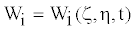Regional dimension-less plate displacement in the domain of region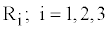a,b Plate dimension in ζ ,η directions respectively

x, y Plate coordinates

t Time

ζ ,η Plate dimension-less coordinates;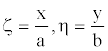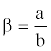Aspect ratio

hi Regional thickness of plate

ρ Plate density

M Number of panel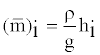Plate mass per unit area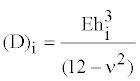Regional flexural rigidity of plate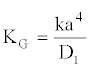Normalized parameter of homogenous sub-grade

ω Natural frequency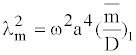Natural frequency parameter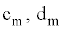Integral values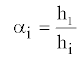Panel thickness ratio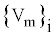Regional nodal vector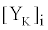Regional transition matrix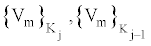Nodal vectors of strip Kj

Φ Restrained cofficient of rotation and translation at ξ = 0,1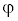Restrained cofficient of rotation and translation at η = 0,1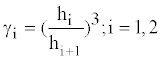Step thickness ratio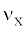Poisson’s ratio

Introduction

A certain structural optimization of a panel may be achieved by possessing a suitable variation of thickness of plate structure. The importance of types of structures increases when the aero-space and underground structures are considered. Survey of the literature on the flexural vibration of thin plate reveals that the work on this topic has been mainly confined to plates with uniform thicknesses. A relatively few studies have been published on the free vibration of isotropic plates of stepped thicknesses. During the past few decades, many researchers were devoted to mathematical modeling and numerical solution for static elastic multi-structure problems. Since vibration analysis of elastic structures plays important roles in engineering applications, this paper is concerned with Finite Strip-Transition Matrix method (FSTM) for vibration analysis of paneled stepped thickness plate.

Although, Chopra  has attempted an exact solution for a simply supported stepped thickness plate with two panels, Warburton  pointed out that continuity conditions used by Chopra  were incorrect. He presented a modified analytical technique for two paneled stepped plate with different properties of orthotropic. Sakata  proposed an approximate formula for estimating the fundamental nature frequency of an isotropic plate with stepped thickness from the natural frequencies of the isotropic plate reduced from the orthotropic one. Recently, Farag  applied a closed form solution for vibrating surfaces of partially restrained and clamped double-panel plates via a power matrix exponential method. Xiang and Wang  also studied the exact vibration solutions of stepped rectangular plates. Xiang et al. [6,7] extended these studies in cooperation with others to include the case of stepped rectangular mindlin plate and the case of stepped circular mindlin plate. Gorman and Singha  outlined the vibration analysis of stepped cantilever plate using a supper position method. A numerical approach for rectangular stepped plate with sides restrained against rotation has been proposed by Laura and Filipich . They ensured that vibration of plate with stepped thicknesses was studied in a very few number of literature. Filipich et al.  used a simple polynomial coordinate function which identically satisfied the restrained boundary conditions of plate with discontinuous thickness. Modal study via a discrete numerical approach, such as finite element method by Mukherjee and Mukhopadhyay , or finite strip method by Cheung and Li [12,13] introduces solution accuracy depending upon the suggested element size. Vibrations of plates with variable thickness were studied by Zanzi and Laura , Gutierrez et al. , Laura and Gutierrez  via different methods. Kaabi and Aksu [17,18] applied a modified method to examine the dynamic behavior of rectangular plates with bilinear variation of thickness. Cheung and Li  achieved a simple finite strip method to analyze the hunched continuous bridges. Vibration of orthotropic rectangular plate with free edges in the case of discontinuously varying thickness was discussed by Laura et al. . They extended their studies to include two other boundary conditions different from Laura et al. . Farag  applied Finite Strip Transition Matrix method to solve the free and forced vibration problems of uniform thickness plate. In extended work, Farag  analyzed the stepped thickness plate analytically by means of the matrix exponential method. Recently, a semi and fully discrete finite element methods for investigating vibration analysis of elastic plate-plate structures are proposed by Junjiam et al. . Semie  explained the numerical modeling of thin plates using the finite element method. Sanches et al.  studied the dynamic stationary response of reinforced plate by the boundary element method.

The present paper offers a modified numerical method relying on reducing the partial differential equation of motion into an ordinary differential one. A convenient basic function is used to obtain the later equation which is solved by Finite Strip Transition Matrix Method (FSTM) inside each panel of plate. Due to the sudden change of thickness between two adjacent panels, continuity conditions must be satisfied to generate the final solution of the entire plate. The known basic function is expressed in a variable parallel to the steps. The reduced differential equation of rectangular stepped plate is still carrying the other unknown variable in the other plate direction. The restrained boundary conditions are included and employed to derive the solution of the particular cases of clamped and simply supported edges.

Equation of Motion

The isotropic rectangular plates with elastically restrained boundary conditions in the two directions ζ and η of plates are considered here. The plate thickness shown in Figure 1 is a uniform in ζ -direction, while it changes suddenly in η -direction at a step Si from hi to hi+1 .

Referring to the domain of the isotropic region Ri , the regional dimensionless partial differential equation of motion of plate vibration is: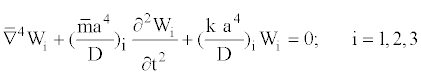(1)

Where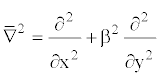The different symbols are denoted in the nomenclatures part where the suffix i means that the mentioned magnitudes are calculated inside the region Ri.

Method of Solution

The partial differential equation (1) is solved numerically under the proposed boundary conditions at (ζ = 0,1) and (η = 0,1) by using the Technique of Finite Strip-Transition Matrix FSTM. In this Technique, the investigated plate is divided into three stepped panels which are separated by a number M-1 of steps. Each individual panel is divided to a number N of finite strips separated by a number N+1 of nodal lines as in Figure 1. The basic function of strip , is applied to reduce the partial differential equation of motion into an ordinary differential one. The reduced differential equation is solved numerically by the FSTM as an initial value problem under the proposed initial boundary conditions at (η = 0) . The initial nodal conditions of each strip are applied to derive the strip end nodal conditions which are used as initial nodal conditions to the next strip. All nodal straining actions of each strip are obtained until the end of the current panel is reached. Because the intermediate end of a current panel is sudden step, the initial nodal conditions of the next panel must be modified by satisfying the compatibility conditions at this step. The FSTM is applied for all strips of all panels until the final boundary end of plate is reached. Satisfying the boundary conditions at (η = 1) , one can obtain the final solution of the equation motion of plate.

Reduction technique

The regional displacement of the stepped plate is: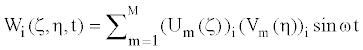(2)

Where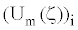is known regional shape function satisfying the plate boundary conditions  at (ζ = 0,1) and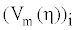is unknown regional longitudinal function to be determined at (η = 0,1) .

Eq. (2) is applied to reduce the partial differentia Eq. (1) Inside the Region Ri i = 1,2,3; into :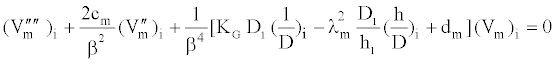(3)

Where,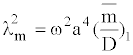,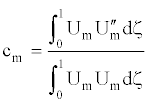,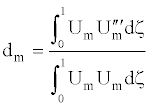Consequently, the equation of motion for any panel becomes: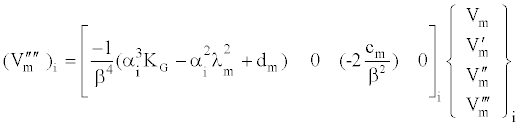(4)

where: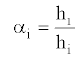is the panel thickness ratio at region Ri i = 1,2,3;

The ordinary differential equation (4) is transformed within a region Ri to a linear system of differential equations expressed as :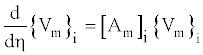(5)

Where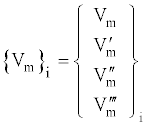(6)

And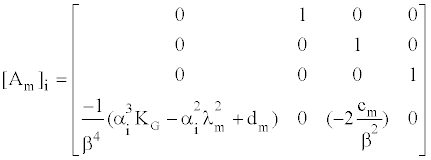(7)

The general solution of the ordinary differential equation is: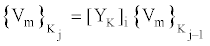(8)

Where δi is the width of strip κj bounded by two nodal lines Kj ,Kj-1 inside region Ri .

The regional Transition Matrix [YK]i is calculated according to the Runge Kutta method such as: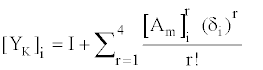(9)

Where I is the unit matrix?

Continuity conditions

The Magnitudes of displacement W slope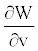Moment MV and shear Qv must satisfy the continuity conditions at the sudden step Si so that: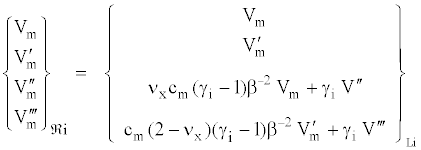(10)

Where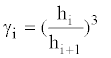is step thickness ratio at step Si ;i=1,2 and νx is Poisson’s ratio.

A right step vector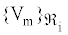of the sudden step Si is achieved by updating the step left vector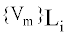due to Eq. (10).

Boundary and initial conditions

Applying the proposed boundary conditions at η = 0 , one can reduce the four initial unknowns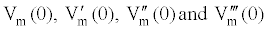to only two unknowns. Consequently, by satisfying the boundary conditions of plate at η = 1 , two characteristic equations for the plate vibration are established. The natural frequency parameters of plate are the Eigen values of the characteristic matrix of these equations. The corresponding Eigen vectors create the mode shapes. Boundary conditions at the edges η = 0,1 are considered for various types of edges , such as simply supported S, clamped C, and free F, elastically restrained against rotation ER . The restrained coefficients of rotation Φ η = 0,1 are usually applied to vary from 0 to ∞.

The initial conditions are expressed as initial valueswhich are the components of initial vector {Vm}o of the first region R1. This initial vector {Vm}o can be derived according to the known boundary conditions at the first nodal edge η = 0 .

Results and Discussions

Different cases of plates composed of different panels with unequal thicknesses and panel widths are investigated by the present technique. This study takes in account the variation of the aspect ratios β and various magnitudes of panel thickness ratio αi i = 1,2,3; and the coefficients of partially restrained boundary conditions. The natural frequency parameter λmn is calculated for every case where Poisson’s ratio is taken as ν = 0.3 .

The natural frequencey coefficients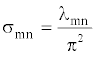based on the present technique are compared as shown in Table 1 with those obtained by Xiang et al.  using an exact solution and using closed form solution .

 SSSS; CSCS; CCCC;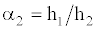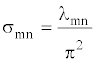Present Xiang et al.  Farag  Present Xiang et al.  Farag  Present s11 02.9015 0 2.9015 02.9015 04.1711 04.1711 04.1711 05.3028 1/2 s12 07.1157 07.1156 07.1156 09.9048 0 9.9047 09.9047 10.5159 s13 13.7849 13.7850 13. 7848 18. 0455 18.0450 18. 0453 18.6238 s11 02.4470 2.4471 02.4470 03.5609 03.5610 03.5609 04.4631 2/3 s12 06.2230 6.2229 06.2229 08.7200 08.7199 08.7199 09.2528 s13 11.9125 11.9480 11.9124 15.6217 15.6210 15.6215 16.0596

Table 1: Comparesons of the natural frequency coefficient σmn for square Plates SSSS and CSCS.

The comparisons are available only for the case of double panel square plate with panel width ratio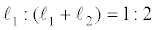as shown in Table 1. Calculations are carried out in two cases of boundary conditions of square plates SSSS and CSCS where S, C mention to the simply supported and clamped edges respectively. The general method of restrained boundary conditions is applied when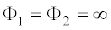,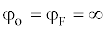to posses the case of full simply supported plate SSSS. Another case of plate CSCS is obtained whereand. The third case is full clamped plate CCCC whereand. The results are obtained for panel thickness ratios equal to 0.5 and 2/3. The comparisons show excellent agreement.

The natural frequencey parameters λmn in the first six modes are obtained in Table 2 for the cases of 3-panels square full clamped plates CCCC with panel width ratio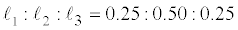. The results are calculated under the variation of α2 from 1.0 to 0.1 where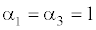. The results show that the natural frequency parametrer inreases by decreasing α2 .

 Natural frequencey parameters λmn α2,α3 α11 α12 α13 α14 α15 α16 1.0, 1.0 35.9195 73.5929 132.3785 211.2511 309.0456 416.1822 0.9, 1.0 37.9563 77.3438 138.6326 222.3554 327.3623 437.8916 0.8, 1.0 40.5830 81.3422 145.6952 236.6180 348.0211 459.2436 0.7, 1.0 43.9586 85.5344 154.3948 236.6180 370.3955 480.2570 0.6, 1.0 46.3017 71.6753 102.2939 255.4175 332.9891 385.8950 0.5, 1.0 54.0737 95.5105 184.6845 267.9179 410.2973 539.8316 0.4, 1.0 62.4249 104.1121 215.7646 343.2175 425.5430 616.2196 0.3, 1.0 76.9445 122.0380 268.1892 366.2887 447.2614 819.1406 0.2, 1.0 109.3091 168.1862 333.4251 376.4200 543.4720 1265.0045 0.1, 1.0 215.0652 311.9320 359.3840 410.0752 1080.9598 2590.7068

Table 2: The natural frequency parameter λmn for 3-panels square clamped plate CCCC.

Table 3 shows the natural frequencey parameters λmn for the cases of 3- panels square full plates CERCER with two opposite edges clamped and other edges ellastically restrained against rotation with restrained coefficient varying from 0 to ∞ . The results are obtained for cases of panel width ratioand α2 varying from 1.0 to 0.25, where. The natural frequencey parameter is obtained for the first three modes. The results show that the natural frequency parametrer decreases by increasing the coefficient of restrained.

 Restraint coefficients against rotation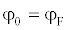α2,α3 λmn 0.0 0.02 0.2 2.0 200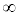1.0, 1.0 α11 35.9195 34.7141 31.1493 29.1644 28.8413 28.8379 α11 73.5929 69.7890 50.1225 55.6106 54.9253 54.9181 α13 132.3787 125.0569 109.4910 103.5695 102.7365 102.7279 0.75, 1.0 α11 42.1654 40.8311 37.1138 35.1818 34.8760 34.7828 α11 83.4136 78.8536 67.9529 63.1631 62.4507 62.4432 α13 149.7636 141.3653 124.3771 118.1902 117.3307 117.3219 0. 5, 1.0 α11 54.0736 52.5033 48.5825 46.7700 46.4956 46.4927 α11 95.5105 90.5687 79.5519 57.0258 74.3679 74.3610 α13 184.8631 175.7379 157.7919 151.4550 150.5831 150.7542 0.25, 1.0 α11 84.6291 88.3274 58.8198 84.7820 84.6307 84.6291 α11 123.5494 135.5536 127.3214 124.0274 123.5543 123.5494 α13 233.6159 282.3131 246.3862 235.1273 233.6312 233.6164

Table 3: The natural frequency parameter λmn for 3-panels square clamped restrained plate CERCER.

This case is carried out for 3-panel square plate, SERSER, simply supported at two opposite edges and partially restrained against rotation at the other edges (Table 4). The results are obtained for panel width ratiosand panel thickness ratio 1 :α2 : 1 where α2 varies from 1.0 to 0.25. Table 4 shows that the natural frequency parameter increases by decreasing the restrained coefficient. For the double panel isotropic, square, clamped supported plate at all edges CCCC the relationships of the variation of natural frequency parameters due to the change of thickness ratio α2 are plotted in figures 2-a,b,c. For the panel width ratio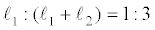, the natural frequency parameters λmn are obtained for the first three modes where m =1, 2 or 3 and n = 1,2 or 3.

 Restraint coefficients against rotationα2,α3 λmn 0.0 0.02 0.2 2.0 2001.0, 1.0 α11 35.9195 34.7141 31.1493 29.1644 28.8413 28.8379 α11 73.5929 69.7890 50.1225 55.6106 54.9253 54.9181 α13 132.3787 125.0569 109.4910 103.5695 102.7365 102.7279 0.75, 1.0 α11 42.1654 40.8311 37.1138 35.1818 34.8760 34.7828 α11 83.4136 78.8536 67.9529 63.1631 62.4507 62.4432 α13 149.7636 141.3653 124.3771 118.1902 117.3307 117.3219 0. 5, 1.0 α11 54.0736 52.5033 48.5825 46.7700 46.4956 46.4927 α11 95.5105 90.5687 79.5519 57.0258 74.3679 74.3610 α13 184.8631 175.7379 157.7919 151.4550 150.5831 150.7542 0.25, 1.0 α11 84.6291 88.3274 58.8198 84.7820 84.6307 84.6291 α11 123.5494 135.5536 127.3214 124.0274 123.5543 123.5494 α13 233.6159 282.3131 246.3862 235.1273 233.6312 233.6164

Table 4: The natural frequency parameter λmn for 3-panels square simply supported- restrained plate SERSER.

Figure 2a: Relationships of the variation of natural frequency parameters λmn due to the change of thickness ratio α2 for the first three modes where m =1 and n =1, 2 or 3 for CCCC case.

Figure 2b: Relationships of the variation of natural frequency parameters λmn due to the change of thickness ratio α2 for the first three modes where m =2 and n =1, 2 or 3 for CCCC case.

Figure 2c: Relationships of the variation of natural frequency parameters λmn due to the change of thickness ratio α2 for the first three modes where m =3 and n =1, 2 or 3 for CCCC case.

Similarly, the case of square isotropic stepped plate CSCS with two opposite edges clamped and other edges simply supported is carried out as shown in figures 3-a,b,c. The frequency parameters λmn are recorded for the case of panel width ratio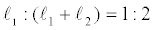when the thickness ratio α2 changes from 0.1 to 1.0. The results show that the natural frequency parameters increase by decreasing the thickness ratio α2 for all modes.

Figure 3a: Relationships of the variation of natural frequency parameters λmn due to the change of thickness ratio α2 for the first three modes where m =1 and n =1, 2 or 3 for CSCS case.

Figure 3b: Relationships of the variation of natural frequency parameters λmn due to the change of thickness ratio α2 for the first three modes where m =2 and n =1, 2 or 3 for CSCS case.

Figure 3c: Relationships of the variation of natural frequency parameters λmn due to the change of thickness ratio α2 for the first three modes where m =3 and n =1, 2 or 3 for CSCS case.

The case of full clamped stepped rectangular plate CCCC with thickness ratio α2=.5, panel width ratioand aspect ratio β of the intire plate varying from 1 to 2 is investigated as shown in figures 4-a,b,c. The resuls show that the parameter λmn increases by increasing the aspect ratio β for all recorded modes.

Figure 4a: Relationship of the variation of natural frequency parameters λmn due to the change of aspect ratio ß for the first two modes where m =1 and n =1, 2 or 3 for CCCC case

Figure 4b: Relationship of the variation of natural frequency parameters λmn due to the change of aspect ratio ß for the first two modes where m =2 and n =1, 2 or 3 for CCCC case

Figure 4c: Relationship of the variation of natural frequency parameters λmn due to the change of aspect ratio ß for the first two modes where m =3 and n =1, 2 or 3 for CCCC case

Conclusion

The finite strip transition matrix method FSTM described here involvoes a numerical solution of stepped paneled plate with classical and restrained boundary conditions. This method is a combination between the strip and transition matrix method to solve the vibration problem of stepped plates as an initial value proplem. Transition matrix method is a semi analytical method relying on estmiating the numerical solution of the intial value problem by means of Range Kutta method. The plate domain is divided into paneled regoins consisting of strips bounded by nodal lines. Each strip is governed by the transition matrix formula which transite from one strip to another via nodal vectors until the final edge is reached. Several cases of double and triple panel plates are investigated for the variation of thickness ratio, aspect ratio, panel width ratio and boundary conditions. To show the accuracy of the present method, the results have been summerized and compared with those obtained by other methods. A good exteremely agreement of results is found for all compared cases.

References

Select your language of interest to view the total content in your interested language

Article Usage

• Total views: 12202
• [From(publication date):
July-2012 - Jun 17, 2019]
• Breakdown by view type
• HTML page views : 8420Can't read the image? click here to refresh